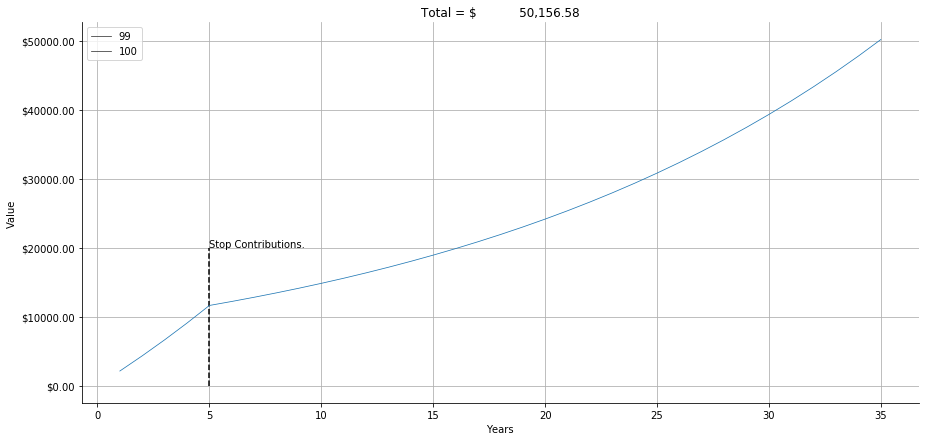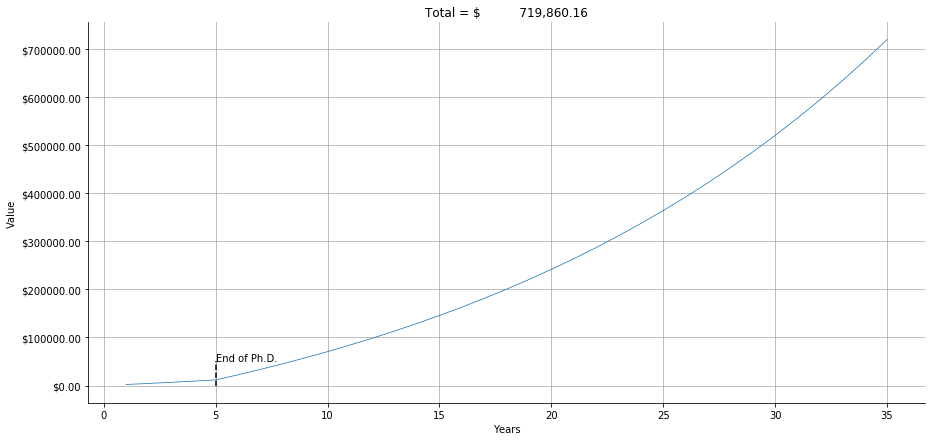# How Compound Interest Can Change Your Life¶

## Some resources.¶

In :
import matplotlib.pyplot as plt
import seaborn as sns
import numpy as np
import pandas as pd


#### This is a quick and dirty function for calculating compound interest¶

In :
# Python3 program to find compound
# interest for given values.

def compound_interest(principle, rate, years, monthly_cont, num_months):
"""
principle = the amount of money that you begin with
rate = the PERCENTAGE OF INTEREST (0-100) that you earn each time
years = the number of times you will gain interest (years)
monthly_contr = the amount you plan to input every month
num_months = number of months of contributions
"""

amount = principle
yearly_contr = monthly_cont * num_months
indiv_amounts = []

# Calculates compound interest
for ii in range(years):
amount += yearly_contr                                                     # Add a single years total contributions
year_interest = amount * (rate/100)                                        # Calculate the interest on the new amount
amount += year_interest                                                    # Add the interest
indiv_amounts.append(amount)                                               # Collect the yearly amounts for later plotting
total_cmp_int = amount - (principle + (monthly_cont * num_months * years))     # Calculate the total compound interest by subtracting the principle and total contributions

# Print info.
print("Compound interest = ${:,.2f}".format(total_cmp_int)) print("Total after {} years =${:,.2f}".format(years,amount))

# return the final amount and individual amounts after each year
return amount, indiv_amounts


# If we assume that we want to try and utilize IU's 457b retirement plan resource, how might this affect our retirement?¶

Total after 30 years = $50,156.58  ### The function outputs a list of each value at the end of each year, I put them together here¶ In : # Combine the yearly amounts into one list all_yearlys = yearlys + yearlys2  ### Create a dataframe for easier plotting¶ In : # Create a dataframe with years and values yearly_values = pd.DataFrame({"Years" : np.arange(1,len(all_yearlys)+1,1), "Value" : all_yearlys })  In : yearly_values.head()  Out: Years Value 0 1 2101.050000 1 2 4306.102500 2 3 6621.407625 3 4 9052.478006 4 5 11605.101907 ### Show how your money grows over time.¶ In : import matplotlib as mpl plt.figure(figsize=(15,7)) ax = sns.lineplot(data = yearly_values, x = "Years", y = "Value", markers = "o", size=100) plt.vlines(x = 5, ymax=20000, ymin=0, linestyles="--") plt.title('Total =${:20,.2f}'.format(yearly_values.iloc[-1]["Value"]))

plt.annotate(s = "Stop Contributions.",
xy = (5,20000))

formatter = mpl.ticker.FormatStrFormatter('$%1.2f') ax.yaxis.set_major_formatter(formatter) plt.grid() sns.despine()### Now, lets assume that we're normal people and we keep making money and we keep putting that money into our account overtime - which also continues to generate compound interest.¶ • Here, we calculate compound interest for contributing$200 every month for the next 35 years (5 Ph.D. years + 30 more, like last time)
In :
# First 5 years in PhD
total_value, yearlys = compound_interest(principle = 1,
rate = 5,
years = 5,
monthly_cont = 200,
num_months = 10) # We don't get paid over the summer

# 200 for 30 more years
total_value, yearlys2 = compound_interest(principle = total_value,
rate = 5,
years = 30,
monthly_cont = 200,
num_months = 12) # Now we do get paid over the summer

all_yearlys = yearlys + yearlys2

# Create a dataframe with years and values
yearly_values = pd.DataFrame({"Years" : np.arange(1,len(all_yearlys)+1,1),
"Value" : all_yearlys
})

Compound interest = $1,604.10 Total after 5 years =$11,605.10
Compound interest = $133,977.38 Total after 30 years =$217,582.48


Total after 5 years = $11,605.10  ### Assuming you get a data science job after you graduate, the average base salary in the US = 122K¶ ### But lets be cautious and assume that we make around$100K for the rest of our lives¶

In :
# 100k / 12 months --> REMEMBER THESE ARE PRETAX CONTRIBUTIONS, SO WE DON'T NEED TO REMOVE INCOME TAX
monthly_payment = 100000 / 12
monthly_payment * .1

Out:
833.3333333333335

Total after 30 years = $719,860.16  ### Combine the first five and last thirty years...¶ In : # Combine the yearly amounts into one list all_yearlys = yearlys + yearlys2  In : # Create a dataframe with years and values yearly_values = pd.DataFrame({"Years" : np.arange(1,len(all_yearlys)+1,1), "Value" : all_yearlys })  In : yearly_values.head()  Out: Years Value 0 1 2101.050000 1 2 4306.102500 2 3 6621.407625 3 4 9052.478006 4 5 11605.101907 ### And what this looks like now is...¶ # You have more than$700k.¶

In :
import matplotlib as mpl
plt.figure(figsize=(15,7))

ax = sns.lineplot(data = yearly_values,
x = "Years",
y = "Value",
markers = "o",
size=100, legend=False)

plt.vlines(x = 5,
ymax=50000,
ymin=0,
linestyles="--")

plt.annotate(s = "End of Ph.D.",
xy = (5,50000))

plt.title('Total = ${:20,.2f}'.format(total)) formatter = mpl.ticker.FormatStrFormatter('$%1.2f')
ax.yaxis.set_major_formatter(formatter)

plt.grid()
sns.despine()### Not everyone is going to be able to create a business that makes them rich. This is how average people accumulate wealth and, fortunately, it's extremely easy. Start as early as possible and stay consistent.¶

In [ ]:
s